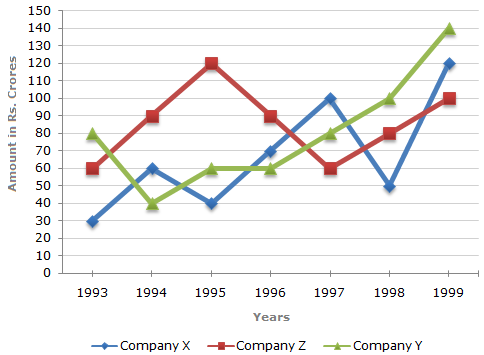# Data Interpretation - Line Charts - Discussion

Discussion Forum : Line Charts - Line Chart 1 (Q.No. 5)
Directions to Solve

Study the following line graph and answer the questions.

Exports from Three Companies Over the Years (in Rs. crore)5.
In how many of the given years, were the exports from Company Z more than the average annual exports over the given years?
2
3
4
5
Explanation:

Average annual exports of Company Z during the given period

 = 1 x (60 + 90 + 120 + 90 + 60 + 80 + 100) 7

 = Rs.600crores 7

= Rs. 85.71 crores.

From the analysis of graph the exports of Company Z are more than the average annual exports of Company Z (i.e., Rs. 85.71 crores) during the years 1994, 1995, 1996 and 1999, i.e., during 4 of the given years.

Discussion:
18 comments Page 1 of 2.

Champ said:   2 years ago
It will be 5 so the answer will be D.

Here, the average you have taken is wrong. For this question average of z is not required but an average of the three companies is required so the answer would be option D 5.
(1)

Rai said:   2 years ago
Z's Average = 600/7 = 85.71.
So, in the year
1994 = 90 , (90>85.71).
1995 = 120,(120>85.71).
1996 = 90,(90>85.71).
1999 = 100,(100>85.71).

Above 4years has more exports than average.

Remaining years were less than average.
i.e 1993 = 60 ,(60<85.71).
1997 = 60,(60<85.71).
1998 = 80,(80<85.71).

Nagendra said:   3 years ago
In majority questions explanation is not right. 93 is less that Z average why 93 is not considered?

Java said:   4 years ago
@Mohan.

Thanks for explaining it.

Aish said:   4 years ago
Thanks for the good explanation @Mohan.

Somya gupta said:   5 years ago
How can you find this?

Then, in 1993 exports are 60 , which is less than 85.71.
in 1997 exports are 60, which is less than 85.71.
in 1998 exports are 80, which is less than 85.71.

Quwi said:   5 years ago

Guesi said:   5 years ago
How the avg of 85.7 is more than 100 In the year 1999?

Or the answer should be 5 if we will compare using the average of the 3 companies combined.